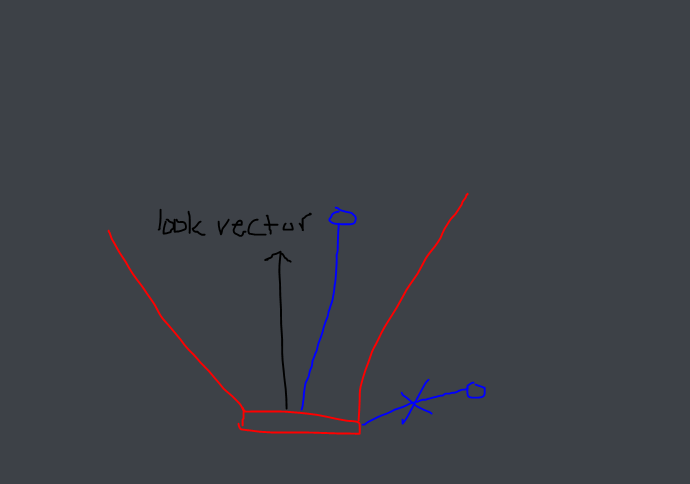# A way to cast one (or multiple) ray(s) to form a angle shape?

So I’m creating a bot that needs to detect the player when in a certain range. Rather than using parts, I’d like to use raycasting to detect this. The only problem is, I don’t know if this type of thing is possible on Roblox yet. Here’s a simple picture of what I want to achieve:

So, is there a way I can achieve this using raycasting? Or should I just weld a part to the block in a similar shape (and use a `.Touched` function to detect the player)?

Quick note, the ray would have to encompass the entire area, not just either side.

1 Like

You can check the positions of each player and figure out if they are in front of the bot by using math(once detected, cast a ray in that direction to see if something is blocking the view from the bot to the player):2 Likes

Pretty much this. You can use the dot product to check if the vector to the player is less than a certain angle to the look vector.

``````local toPlayer = (PlayerPosition - BotPosition).Unit
local look = BotCFrame.LookVector
local dot = look:Dot(toPlayer)
local angle = math.acos(math.clamp(dot, -1, 1))
-- within sight
end
``````
5 Likes

How would I be able to limit how far this will detect the player?

I would personally just add a magnitude check to the above code. For example,

``````local toPlayer = (PlayerPosition - BotPosition).Unit
local mag = (PlayerPosition - BotPosition).magnitude
local look = BotCFrame.LookVector
local dot = look:Dot(toPlayer)
local angle = math.acos(math.clamp(dot, -1, 1))
if angle < math.rad(30) and mag < maxDistance then
-- within sight
end
``````
1 Like